Electrical Engineering

# Electrical Engineering | Electrical Engineering SSC JE (Technical) - Electrical Engineering (EE)

 1 Crore+ students have signed up on EduRev. Have you?

Network Theorems

1. Thevenin's theorem
2. Norton's theorem
3. Superposition theorem
4. Reciprocity theorem
5. Max power transfer theorem
6. Compensation theorem
7. Tellegan's theorem
8. Miliman's theorem
9. Substitution theorem

(1) Thevenin's Theorem : Any two terminal linear bilateral network can be replaced by a voltage source in series with an impedance, the voltage source is the voltage across the open circuited terminals and series impedance is the equivalent inpedance seen across the terminals by replacing all the sources in network with their internal impedances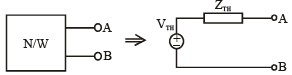VTH → thevenin voltage = VOC across AB.
ZTH → thevenin's equivalent impedance

Norton's theorem ; Any two terminal linear bilateral network containing active and passive elements can be replaced by an equivalent current source in parallel to an equivalent impedance current source is equal to short circuited current through the given terminals and equivalent impedance is the impedance seen across the terminals by replacing all the sources with their internal impedances.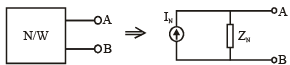IN → Norton's equivalent current

ZN →Norton's equivalent impedance.
Z= ZTH
Here also, there can be three cases as in thevenin's theorem. And, the equivalent ZTH calculation procedure remains same.

Superposition theorem: This theorem states that if a no. of voltage sources or current sources are acting together in an bilateral network then resultant current voltage in any branch is algebraic sum of current / voltage due to individual sources by replacing them with their internal impedances.

Note :
(a) The superposition theorem is applicable to linear time varying and LTI network.
(b) It is also applicable for circuit having intial condition.
(c) It is applicable for linear network.

Example : For the given circuit, the current I is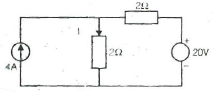(a) 2 A        (b) 5A

(c) 7 A        (d) 9A

Solution : (c) Use superposition theorem.
Due to current source.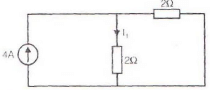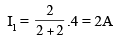Due to voltage source.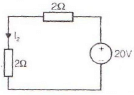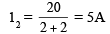Current I = I1 + I2
⇒ I = 2 + 5
⇒ I = 7A

Reciprocity Theorem : This theorem states that in a linear bilateral reciprocal network if I is the current in branch X due to a voltage source V in branch Y then same current will flow in branch Y when the same voltage source is connected in branch X.

Note :

• The initial conditions are assumed to be zero in reciprocity theorem. 
• There should not be any extra dependent of independent sources in network.

Maximum Power Transfer Theorems : The following maximum power transfer theorems determine the values of the load impedances which result in maximum power transfer across the terminals of an active network

We consider a series combination of source and fixed complex impedance delivering power to a load consisting of a variable resistance or a variable  complex impedance

Case 1 : Load is variable resistance RL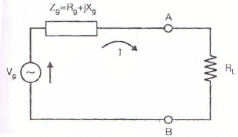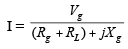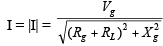Power delivered to RL is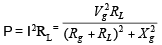To determine the value of R for maximum power transferred to the load,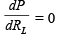We arrive at the condition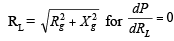Hence with a variable pure resistance load the maximum power is delivered across the terminals of the active network if the load resistance is made equal to the absolute value of the active network impedance

If the reactive component of the impedance in series with source is zero i.e., Xg = 0, then the maximum power is transferred to the load when the load and source resistances are equal i.e., R= Rg.

Case 2 : Load Impedance ZL with variable resistance and variable reactance: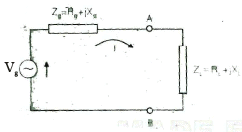For maximum power transfer RL= Rg, XL = –Xg  i.e.Z= Z

With the load impedance consisting of variable resistance and variable reactance, maximum power transfer across the terminals of the active network occurs when the load impedance ZL is equal to the complex conjugate of the network impedance Zg.

Example : An independent voltage source in series with an impedance Zs = Rs + jXdelivers a maximum average power to a load impedance ZL when

(a)ZL = RS + jXs       (b) ZL = Rs

(c)ZL = jXs                   (d) ZL = Rs – jXs

Solution : (D)

Case 3 : Load impedance, ZL with variable resistance and fixed reactance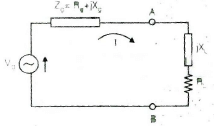For maximum power transfer.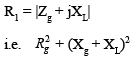Compensation Theorem : The compensation theorem is useful in determining the current and voltage changes in a circuit element when the value of its impedance is changed. This application occurs in bridge and potentiometer circuits where a slight change in one impedance results in shift from the null condition.

Statement : In a linear time invariant bilateral network if the resistance R of a branch is changed by ΔR then the currents in all branch of the network will change and this change in current can be lound by connecting a voltage source vc such that VC = iΔR in series with resistance (R + ΔR)

Here the current i represent current through R before change and all sources are replaced by their internal impedance to find out the change in current.

Tellegan's theorem : This theorem states that the algebraic sum of power consumed and delivered by each branch of a network is always zero.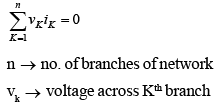• It also holds for the Laplace transfors of the branch voltages and branch currents

we can write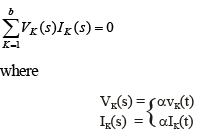Millman's theorem

Consider the circuit in Fig. where several voltage sources V1, V2,....., Vn having internal impedances Z1, Z2,...., Zn are connected in parallel supplying a common load ZL. Millman's theorem states that this arrangement can be replaced by a single voltage source Veq in series with an impedance Zeq as shown.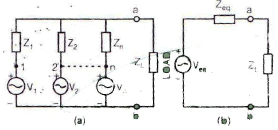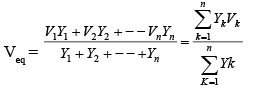and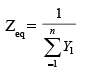The document Electrical Engineering | Electrical Engineering SSC JE (Technical) - Electrical Engineering (EE) is a part of the Electrical Engineering (EE) Course Electrical Engineering SSC JE (Technical).
All you need of Electrical Engineering (EE) at this link: Electrical Engineering (EE)

## Electrical Engineering SSC JE (Technical)

2 videos|75 docs|40 tests

## Electrical Engineering SSC JE (Technical)

2 videos|75 docs|40 tests

Track your progress, build streaks, highlight & save important lessons and more!(Scan QR code)

,

,

,

,

,

,

,

,

,

,

,

,

,

,

,

,

,

,

,

,

,

;